PageRank Algorithm
10/1/2022 by Junxiao Guo

## Introduction

PageRank is an algorithm used by Google to rank web pages in its search engine search results. The algorithm is named after Larry Page, one of the founders of Google. Google's search engine uses it to analyze the relevance of web pages. And importance is often used in search engine optimization as one of the factors to evaluate the effectiveness of web page optimization.

Google uses PageRank to make those webpages with more "rank/importance" improve the ranking of the website in the search results, thereby improving the relevance and quality of the search results. The higher the PR value (how to calculate it will be described below), the higher the page is The more popular. Currently, PageRank is no longer the only algorithm Google uses to rank web pages, but it is the earliest and most famous algorithm.

PageRank is a link analysis algorithm. It achieves the purpose of "measure the relative importance of an element in the collection" by assigning numerical weights to the elements in the hyperlink collection, and PageRank can use any element with A collection of entities that refer to each other.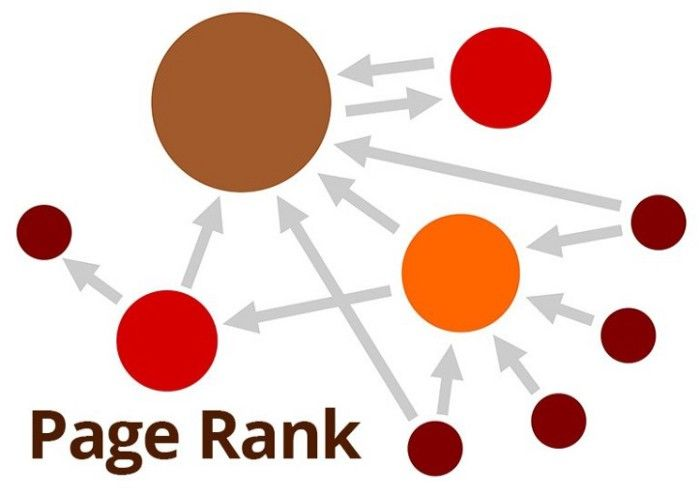## Algorithm

The following introduction takes the event of a user clicking on a web link as the background

In general, PageRank uses a probability distribution to indicate the probability that a user randomly clicks on a link (the total probability of clicking on all links is 1). Before starting the calculation, the total probability will be evenly distributed to each file, making the set The probability of all files being accessed is equal. In the next iteration, the algorithm will continuously adjust the PR value according to the actual situation of the data.

### Basic algorithm ideas

Assume a set of 4 web pages: A, B, C, and D. Multiple links pointing to the same page on the same page are regarded as the same link, and the initial PageRank value of each page is the same. The original algorithm sets the initial value of each page to 0.25 (probability average distribution for 4 pages).

If all pages are connected to A, then the PR value of A becomes the sum of the PR values ​​of B, C, and D: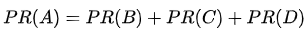If B is linked to A and C, C is linked to A, and D is linked to A, B, C. There is only one vote in total for the first page. So B gives A and C half votes for each page. By analogy, only one-third of D's votes are added to A's PR value: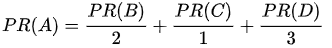Using a mathematical formula to express the above scenario, the total number of times that each page is directed to other web pages $L(x)$ score the PR value of the page and add it to the page pointed to: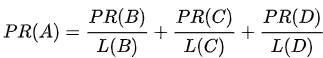Finally, all these PR values ​​are converted into percentages and multiplied by a correction coefficient $d$. Since the PR value passed by "webpages without external links" will be 0, and this will recursively cause the calculation of the PR value of the pages that point to it to also be zero, so assign each page a minimum value (1- d)/N: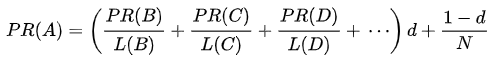In the end, the PR value of a page will be used as an indicator of the search engine's ranking of the page.

In order to optimize the algorithm, the concept of random surfer is introduced. It is assumed that someone randomly clicks on certain pages and links. Assuming that the user keeps clicking on the link until he enters a web page without external links, at this time he will randomly click on other web pages.

A webpage without external links will swallow the user's probability of continuing to browse down. In order to solve this problem, it is assumed that the page will link to all webpages in the combination (regardless of whether it is relevant), and make the PR value of such a webpage equal to all webpages. This probability is called the residual probability (residual probability).

After that, the damping factor $d$(damping factor) is introduced. Assuming that the coefficient is 0.85, it represents the probability that the user will continue to visit a page at any time. Correspondingly, 0.15 means that the user will no longer continue. Browsing, but the probability of starting to browse a new web page randomly.

Therefore, for page i, the calculation formula of the PR value is: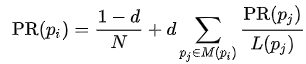Here, p1,p2,...,pn is the target page pi, M(pi) is the set of pages, L(pj) is the number of pages linked out, and N is the number of all pages

## shortcoming

The ranking of the old page tends to be higher than that of the new page, because even a high-quality new page often does not have many links, unless it is a sub-site of an existing site